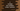# C program to multiply two numbers using plus or addition operator## C program to multiply two numbers using plus or addition operator:

In this post, we will learn how to multiply two numbers by using plus or addition operator. We will not use the multiplication operator *, but instead we will use +.

For example, if we are multiplying two numbers 4 and 3, we are actually adding 4 for 3 times or 3 for 4 times.

i.e. 4*3 is equal to 4 + 4 + 4 or 3 + 3 + 3 + 3.

To do this programmatically, you need to use a loop. The loop will keep adding the number repeatedly to find the result. We will also take the numbers as inputs from the user.

With this program, you will learn how to take user inputs, how to use loops, how to use the addition operator to find out the multiplication and how to print data to the user.

### Algorithm:

We will use the below algorithm:

• Take the numbers as inputs from the user.
• Run one loop for the smaller number of times. We will run it for smaller number of times to make the loop smaller. For example, if we are multiplying 2 with 1000, the loop will run for 2 times, not 1000 times.
• Initialize one variable as 0 to hold the final multiplication.
• Inside the loop, keep adding the other variable to the multiplication variable.
• Once the loop is completed, print out the result.

### C program:

Below is the complete C program:

``````#include <stdio.h>

int main()
{
int first, second, i;
int smaller, bigger;
int multiplication = 0;

printf("Enter the first number: ");
scanf("%d", &first);

printf("Enter the second number: ");
scanf("%d", &second);

if (first != 0 || second != 0)
{
if (first < second)
{
smaller = first;
bigger = second;
}
else
{
smaller = second;
bigger = first;
}

for (i = 0; i < smaller; i++)
{
multiplication += bigger;
}
}

printf("Multiplication: %d\n", multiplication);

return 0;
}``````

### Explanation:

Here,

• first and second are two integer variables to hold the first and the second numbers.
• i is initialized to use in the loop.
• smaller and bigger are two integer variables to hold the smaller and larger values.
• multiplication is to hold the final multiplication.
• If any of these number is 0, it will not try to find anything, because the multiplication will be zero.
• It is reading the numbers as inputs from the user. The if-else block is finding the smaller and larger number among these two integers.
• The for loop is finding the multiplication by adding the bigger value to the multiplication variable.
• Once the loop is complete, it prints the multiplication value.

### Sample output:

If you run this program, it will print output as like below:

``````Enter the first number: 2
Enter the second number: 2000
Sum: 4000

Enter the first number: 10
Enter the second number: 2345
Sum: 23450

Enter the first number: 1234
Enter the second number: 0
Sum: 0``````

### C program example to find the multiplication using plus with a while loop:

The above program uses a for loop. We can also use a while loop to find the multiplication.

For example:

``````#include <stdio.h>

int main()
{
int first, second, i = 0;
int smaller, bigger;
int multiplication = 0;

printf("Enter the first number: ");
scanf("%d", &first);

printf("Enter the second number: ");
scanf("%d", &second);

if (first != 0 || second != 0)
{
if (first < second)
{
smaller = first;
bigger = second;
}
else
{
smaller = second;
bigger = first;
}

while (i < smaller)
{
multiplication += bigger;
i++;
}
}

printf("Multiplication: %d\n", multiplication);

return 0;
}``````

This example uses a while loop. The loop runs until the value of i is smaller than the smaller number. Inside the loop, we are adding the bigger number to the final result value. This part is similar to the previous example.

If you run this program, it will print similar output.